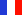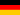###### Descriptions and Examples for the POV-Ray Raytracerby Friedrich A. Lohmüller Loops, Sine, Cosine and POV-Ray
ItalianoFrançaisDeutschHome
- POV-Ray Tutorial
Loops,
Sine, Cosine
and POV-Ray
1. Linear Waves
>2. Concentric waves
3. Flying Carpets

## Sine and Cosine going round in loops- concentric waves -

Until now we applied sine function only in x direction. A very charming effect will appear if we use a sine modultion to the y position of each sphere depending from here distance of the center. The distance of a point from the origin in cartesian coordinates is calculated by calculating the square root of sum of the sqare of the X value plus the square of Z value - as Pythagoras found out somewhat before.

 ```#declare Ball = sphere{<0,0,0>,0.25 texture{ pigment{color rgb<1,0.65,0.0>} finish {diffuse 0.9 phong 1} }// end of texture }// end of sphere #declare Z = -5; // start value Z #declare EndZ = 5;// end value Z #declare Step = 0.5;// step value //----------- loop start Z : #while ( Z < EndZ + Step) #declare X = -5; // start value X #declare EndX = 5; // end value X //------------ loop start X: #while ( X < EndX + Step) // calculazione // della distanza dall'origine: #declare R = sqrt(X*X + Z*Z); // modulato dipendente di R: object{ Ball translate < X, sin(2*R),Z>} #declare X = X+Step;//next X value #end // ---------------- loop end X #declare Z = Z+Step;//next Z value #end // --------------- loop end Z ```Modified with
 ```#declare R = sqrt( X*X + Z*Z); #if (R < 5) object{Ball translate} #end```
we limitate the distance from the y-axis on R < 5 and use cos(A) instead of the sine:Reducing the radius and increasing the density of the spheres will increase the number of objects to calculate immense - in the following scenery there are about 20000 spheres in game and it needs about 14 MB RAM to caculate it. In addition the y-value of the spheres was modulated antiproportional to the distance R from the central y-axis.

 ```#declare Ball = sphere{<0,0,0>,0.25 texture{ pigment{color rgb<1,0.65,0.0>} finish {diffuse 0.9 phong 1} }// end of texture }// end of sphere #declare E = 8; #declare Z = -E; // start value Z #declare EndZ = E; // end value Z #declare Step = 0.1;// step value //------ loop start Z: #while ( Z < EndZ + Step) #declare X = -E; // start value X #declare EndX = E; // end value X //------- loop start X: #while ( X < EndX + Step) #declare R = sqrt( X * X + Z * Z); #if (R < E) object{ Ball translate} #end // of "#if (R < E)" #declare X = X+Step;// next X value #end // ---------------- loop end X #declare Z = Z+Step;// next Z value #end // ---------------- loop end Z```top

 © Friedrich A. Lohmüller, 2011 www.f-lohmueller.de